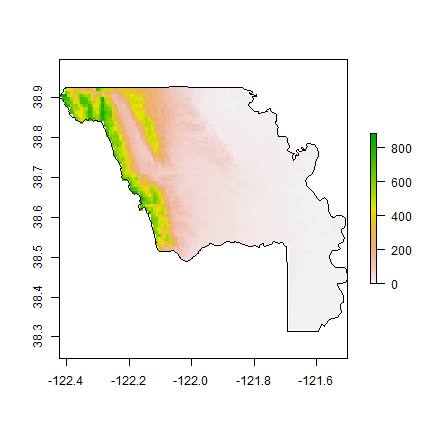Map overlay¶

Introduction¶

This document shows some example R code to do “overlays” and associated spatial data manipulation to accompany Chapter 11 in O’Sullivan and Unwin (2010). You have already seen many of this type of data manipulation in previsous labs. And we have done perhaps more advanced things using regression type models (including LDA and RandomForest). This lab is very much a review of what you have already seen: basic spatial data operations in R. Below are some of the key packages for spatial data analysis that we have been using.

sp - Defines classes (data structures) for points, lines, polygons, rasters, and their attributes, and related funcitons for e.g, plotting. The main classes are SpatialPoints, SpatialLines, SpatialPolygons, SpatialGrid (all also with ‘DataFrame’ appended if the geometries have attributes)

raster - Defines alternative classes for raster data (RasterLayer, RasterStack, RasterBrick) that can be used for very large data sets. The package also provides many functions to manipulate raster data. It also extends to sp and rgeos packages for manipulating vector type data

rgdal - Read or write spatial data files (raster uses it behind the scenes).

rgeos - Geometry manipulation for vector data (e.g. intersection of polygons) and related matters.

spatstat - The main package for point pattern analysis (here used for a density function).

Get the data¶

You can get the data for this tutorial with the “rspatial” package that you can install with the line below.

if (!require("rspatial")) devtools::install_github('rspatial/rspatial')

Selection by attribute¶

By now, you are well aware that in R, polygons and their attributes can be represented by a ‘SpatialPolygonsDataFrame’ (a class defined in the sp package). Here we use a SpatialPolygonsDataFrame of California counties.

library(rspatial)
library(raster)
counties <- sp_data('counties.rds')

Selection by attribute of elements of a SpatialPolygonsDataFrame is similar to selecting rows from a data.frame. For example, to select Yolo county by its name:

yolo <- counties[counties$NAME == 'Yolo', ] plot(counties, col='light gray', border='gray') plot(yolo, add=TRUE, density=20, lwd=2, col='red')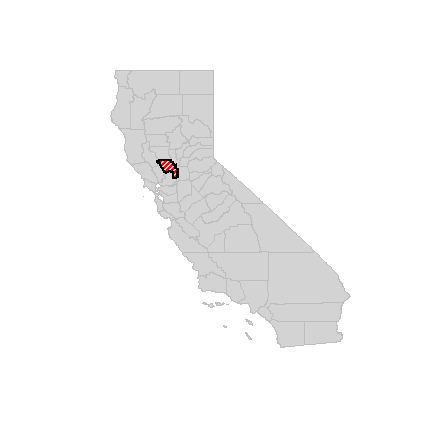You can interactively select counties this way: plot(counties) s <- select(counties) Intersection and buffer¶ I want to select the railroads in the city of Davis from the railroads in Yolo county. First read the data, and do an important sanity check: are the coordinate reference systems (“projections”) the same? rail <- sp_data('yolo-rail.rds') rail ## class : SpatialLinesDataFrame ## features : 4 ## extent : -122.0208, -121.5089, 38.31305, 38.9255 (xmin, xmax, ymin, ymax) ## crs : +proj=longlat +datum=NAD83 +no_defs +ellps=GRS80 +towgs84=0,0,0 ## variables : 1 ## names : FULLNAME ## min values : Abandoned RR ## max values : Yolo Shortline RR # removing attributes that I do not care about rail <- geometry(rail) class(rail) ##  "SpatialLines" ## attr(,"package") ##  "sp" city <- sp_data('city.rds') class(city) ##  "SpatialPolygonsDataFrame" ## attr(,"package") ##  "sp" city <- geometry(city) class(city) ##  "SpatialPolygons" ## attr(,"package") ##  "sp" projection(yolo) ##  "+proj=longlat +datum=NAD83 +no_defs +ellps=GRS80 +towgs84=0,0,0" projection(rail) ##  "+proj=longlat +datum=NAD83 +no_defs +ellps=GRS80 +towgs84=0,0,0" projection(city) ##  "+proj=lcc +lat_1=38.33333333333334 +lat_2=39.83333333333334 +lat_0=37.66666666666666 +lon_0=-122 +x_0=2000000 +y_0=500000.0000000001 +datum=NAD83 +units=us-ft +no_defs +ellps=GRS80 +towgs84=0,0,0" Ay, we are dealing with two different coordinate reference systems (projections)! Let’s settle for yet another one: Teale Albers (this is really the “Albers Equal Area projection with parameters suitable for California”. This particular set of parameters was used by an California State organization called the Teale Data Center, hence the name. library(rgdal) TA <- CRS("+proj=aea +lat_1=34 +lat_2=40.5 +lat_0=0 +lon_0=-120 +x_0=0 +y_0=-4000000 +datum=NAD83 +units=m +ellps=GRS80") countiesTA <- spTransform(counties, TA) yoloTA <- spTransform(yolo, TA) railTA <- spTransform(rail, TA) cityTA <- spTransform(city, TA) Another check, let’s see what county Davis is in, using two approaches. In the first one we get the centroid of Davis and do a point-in-polygon query. dav <- coordinates(cityTA) davis <- SpatialPoints(dav, proj4string=TA) over(davis, countiesTA) ## STATE COUNTY NAME LSAD LSAD_TRANS ## 0 06 113 Yolo 06 County An alternative approach is to intersect the two polygon datasets. i <- intersect(cityTA, countiesTA) data.frame(i, area=area(i, byid=TRUE)) ## STATE COUNTY NAME LSAD LSAD_TRANS area ## 1 06 113 Yolo 06 County 25633905.4 ## 2 06 095 Solano 06 County 78791.6 plot(cityTA, col='blue') plot(yoloTA, add=TRUE, border='red', lwd=3)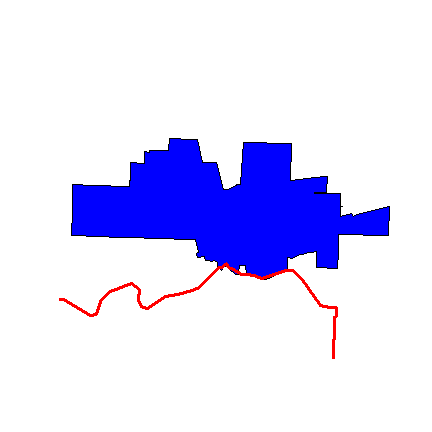So we have a little sliver of Davis inside of Solano. Everything looks OK. Now we can intersect rail and city, and make a buffer. davis_rail <- intersect(railTA, cityTA) Compute a 500 meter buffer around railroad inside Davis: buf <- buffer(railTA, width=500) rail_buf <- intersect(buf, cityTA) plot(cityTA, col='light gray') plot(rail_buf, add=TRUE, col='light blue', border='light blue') plot(railTA, add=TRUE, lty=2, lwd=6) plot(cityTA, add=TRUE) plot(davis_rail, add=TRUE, col='red', lwd=6) box()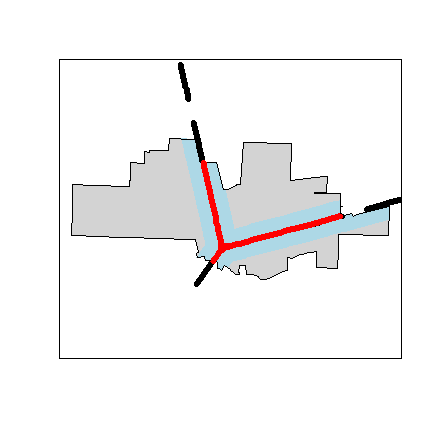What is the percentage of the area of the city of Davis that is within 500 m of a railroad? round(100 * area(rail_buf) / area(cityTA)) ##  31 Proximity¶ Which park in Davis is furthest, and which is closest to the railroad? First get the parks data. parks <- sp_data('parks.rds') proj4string(parks) ##  "+proj=lcc +lat_1=38.33333333333334 +lat_2=39.83333333333334 +lat_0=37.66666666666666 +lon_0=-122 +x_0=2000000 +y_0=500000.0000000001 +datum=NAD83 +units=us-ft +no_defs +ellps=GRS80 +towgs84=0,0,0" parksTA <- spTransform(parks, TA) Now plot the parks that are the furthest and the nearest from a railroad. plot(cityTA, col='light gray', border='light gray') plot(railTA, add=T, col='blue', lwd=4) plot(parksTA, col='dark green', add=TRUE) d <- gDistance(parksTA, railTA, byid=TRUE) dmin <- apply(d, 2, min) parksTA$railDist <- dmin
i <- which.max(dmin)
data.frame(parksTA)[i,]
##              PARK     PARKTYPE            ADDRESS railDist
## 25 Whaleback Park NEIGHBORHOOD 1011 MARINA CIRCLE 4330.938
plot(parksTA[i, ], add=TRUE, col='red', lwd=3, border='red')
j <- which.min(dmin)
data.frame(parksTA)[j,]
##                    PARK     PARKTYPE         ADDRESS railDist
## 13 Toad Hollow Dog Park NEIGHBORHOOD 1919 2ND STREET 23.83512
plot(parksTA[j, ], add=TRUE, col='red', lwd=3, border='orange')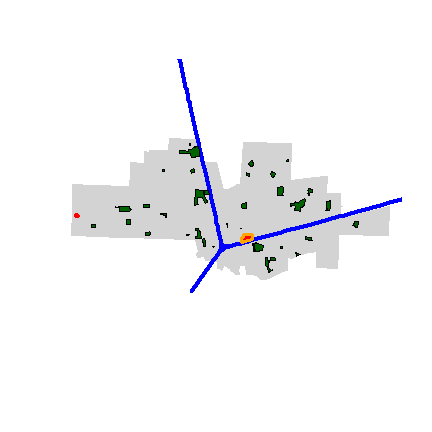Another way to approach this is to first create a raster with distance to the railroad values. Here we compute the average distance to any place inside the park, not to its border. You could also compute the distance to the centroid of a park.

library(raster)
# use cityTA to set the geogaphic extent
r <- raster(cityTA)
# arbitrary resolution
dim(r) <- c(50, 100)
# rasterize the railroad lines
r <- rasterize(railTA, r, field=1)
# compute distance
d <- distance(r)
# extract distance values for polygons
dp <- extract(d, parksTA, fun=mean, small=TRUE)
dp <- data.frame(parksTA$PARK, dist=dp) dp <- dp[order(dp$dist), ]
plot(d)
plot(railTA, add=T, col='blue', lty=2)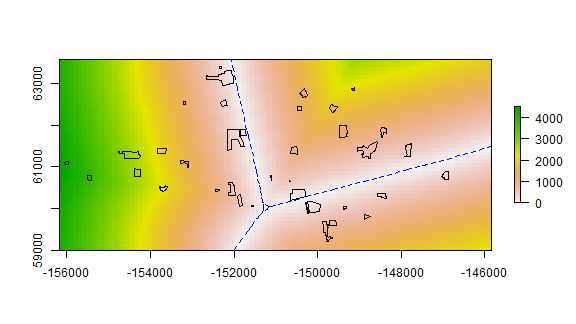Thiessen polygons¶

Here I compute Thiessen (or Voronoi) polygons for the Davis parks. Each polygon shows the area that is closest to (the centroid of) a particular park.

library(dismo)
centroids <- coordinates(parksTA)
v <- voronoi(centroids)
plot(v)
points(centroids, col='blue', pch=20)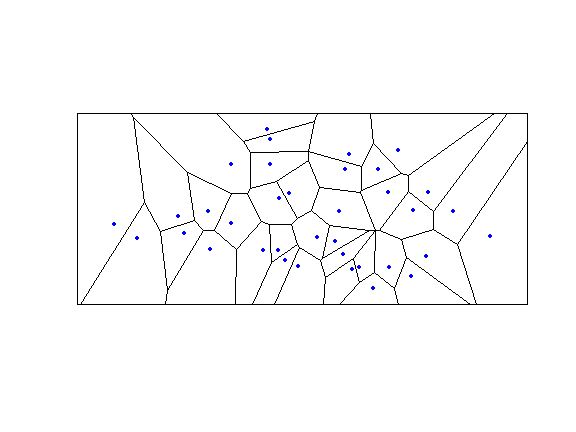To keep the polygons within Davis.

proj4string(v) <- TA
vc <- intersect(v, cityTA)
plot(vc, border='red')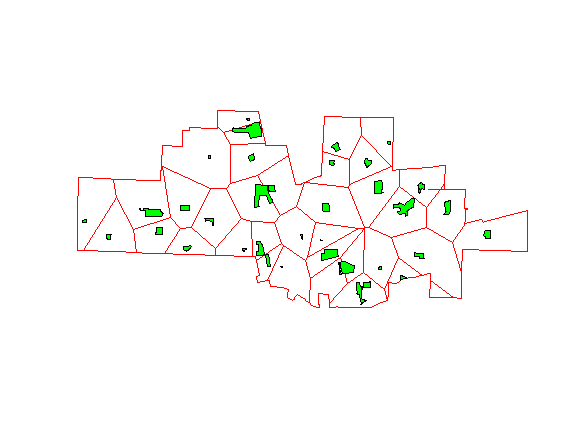Fields¶

Raster data¶

raster data can be read with readGDAL to get a SpatialGridDataFrame. But I prefer to use the raster package to create Raster* objects. Raster* stands for RasterLayer, RasterStack, or RasterBrick. See the vignette for the raster package for more details: http://cran.r-project.org/web/packages/raster/vignettes/Raster.pdf

library(raster)
# get a RasterLayer with elevation data
alt <- sp_data("elevation")
alt
## class      : RasterLayer
## dimensions : 239, 474, 113286  (nrow, ncol, ncell)
## resolution : 0.008333333, 0.008333333  (x, y)
## extent     : -123.95, -120, 37.3, 39.29167  (xmin, xmax, ymin, ymax)
## crs        : +proj=longlat +datum=WGS84 +no_defs +ellps=WGS84 +towgs84=0,0,0
## source     : memory
## names      : elevation
## values     : -30, 2963  (min, max)

The CRS of RasterLayer ‘alt’ is “lon/lat””, and so is the CRS of ‘yolo’. However, ‘yolo’ has a different datum (NAD83) than ‘alt’ (WGS84). While there is no real difference between these, this will lead to errors, so we first transform yolo. It is generally better to match vector data to raster data than vice versa.

yolo <- spTransform(yolo, crs(alt))
plot(alt)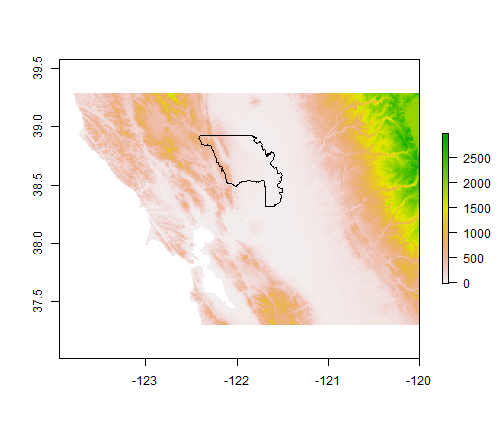Shaded relief is always nice to look at.

slope <- terrain(alt, opt='slope')
aspect <- terrain(alt, opt='aspect')
hill <- hillShade(slope, aspect, 40, 270)
plot(hill, col=grey(0:100/100), legend=FALSE, main='Elevation')
plot(alt, col=rainbow(25, alpha=0.35), add=TRUE)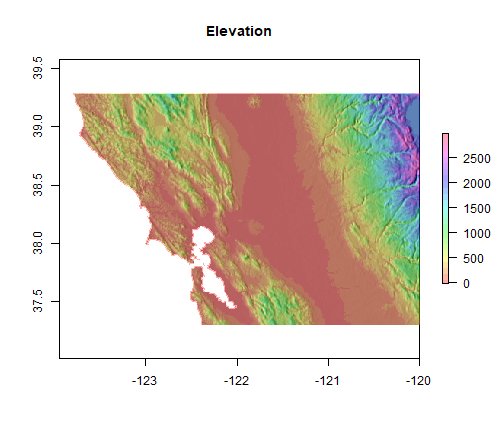You can also try the plot3D function in the rasterVis package.

Query¶

Now extract elevation data for Yolo county.

v <- extract(alt, yolo)
hist(unlist(v), main='Elevation in Yolo county')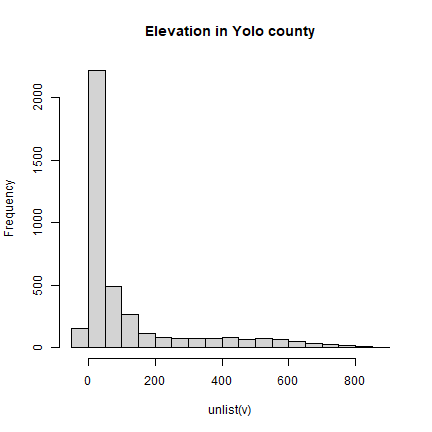Another approach:

# cut out a rectangle (extent) of Yolo
yalt <- crop(alt, yolo)
# 'mask' out the values outside Yolo
# summary of the raster cell values
##         elevation
## Min.           -1
## 1st Qu.         6
## Median         30
## 3rd Qu.       110
## Max.          882
## NA's         4171